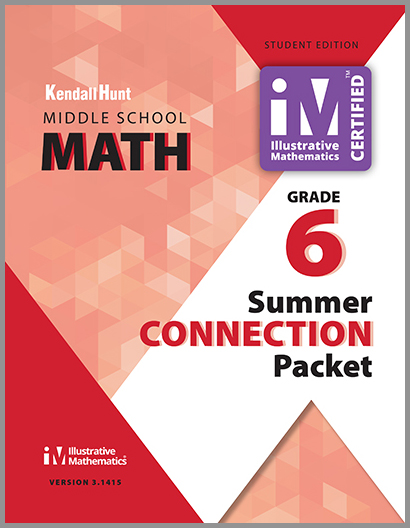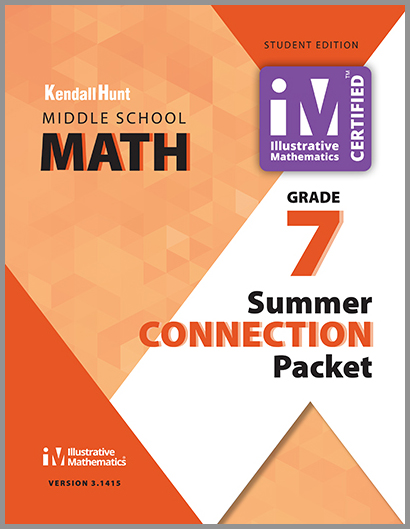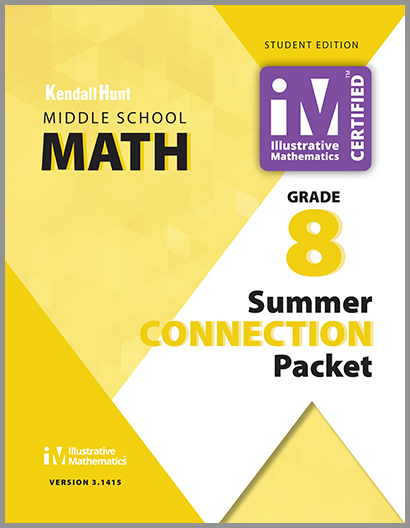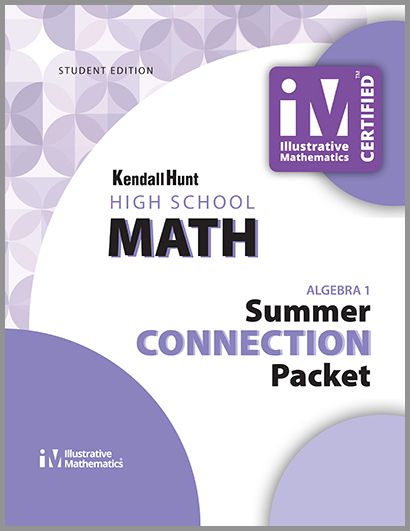### Summer Connection Packets

were created in an effort to support students and ensure they are prepared for in grade level learning by late summer/early fall, feature the most critical math lessons - grade-level content that is considered to be imperative and was missed due to school closures.

Schoolkit will be providing 24 video lessons for IM 6–8 Math and Algebra 1 to be aired on PBS stations later this summer. These videos will help students be prepared in the fall. Please find links to these videos under the corresponding grade level below.

For more information about these Summer Connection Packets, please contact Pam Roth at proth@kendallhunt.com or 1-800-542-6657, ext. 1059.

Illustrative Mathematics Grade 6 Summer Connection Sample.pdf

## Print Materials### GRADE 6

Unit 2: Introducing Ratios

• Lesson 3, Recipes
• Lesson 5, Defining Equivalent Ratios
• Lesson 6, Introducing Double Number Line Diagrams
• Lesson 8, How Much for One?
• Lesson 9, Constant Speed
• Lesson 11, Representing Ratios with Tables
• Lesson 12, Navigating a Table of Equivalent Ratios

Unit 3: Unit Rate and Percentages

• Lesson 4, Converting Units
• Lesson 5, Comparing Speeds and Prices
• Lesson 11, Percentages and Double Number Lines
• Lesson 15, Finding The Percent of That
• Lesson 16, Finding the Percentage

Unit 6: Expressions and Equations

• Lesson 1, Tape Diagrams and Equations
• Lesson 2, Truth and Equations
• Lesson 4, Practice Solving Equations and Representing Situations with Equations
• Lesson 6, Write Expressions Where Letters Stand for Numbers
• Lesson 8, Equal and Equivalent
• Lesson 9, The Distributive Property, Part I
• Lesson 10, The Distributive Property, Part II
• Lesson 16, Two Related Quantities, Part I
• Lesson 17, Two Related Quantities, Part II

Unit 7: Rational Numbers

• Lesson 1, Positive and Negative Numbers
• Lesson 2, Points on the Number Line
• Lesson 3, Comparing Positive and Negative Numbers
• Lesson 6, Absolute Value of Numbers
• Lesson 11, Points on the Coordinate Plane
• Lesson 13, Interpreting Points on a Coordinate Plane
• Lesson 14, Distances on a Coordinate Plane### GRADE 7

Unit 2: Introducing Proportional Relationships

• Lesson 4, Proportional Relationships and Equations
• Lesson 6, Using Equations to Solve Problems
• Lesson 7, Comparing Relationships with Tables
• Lesson 8, Comparing Relationships with Equations
• Lesson 10, Introducing Graphs of Proportional Relationships
• Lesson 11, Interpreting Graphs of Proportional Relationships
• Lesson 12, Using Graphs to Compare Relationships

Unit 5: Rational Number Arithmetic

• Lesson 1, Interpreting Negative Numbers
• Lesson 2, Changing Temperatures
• Lesson 3, Changing Elevation
• Lesson 4, Money and Debts
• Lesson 5, Representing Subtraction
• Lesson 6, Subtracting Rational Numbers
• Lesson 8, Position, Speed, and Direction
• Lesson 9, Multiplying Rational Numbers
• Lesson 11, Dividing Rational Numbers
• Lesson 13, Expressions with Rational Numbers
• Lesson 14, Solving Problems with Rational Numbers

Unit 6: Expressions, Equations and Inequalities

• Lesson 10, Different Options for Solving One Equation
• Lesson 11, Using Equations to Solve Problems
• Lesson 18, Subtraction in Equivalent Expressions
• Lesson 20, Combining Like Terms (Part I)
• Lesson 21, Combining Like Terms (Part II)
• Lesson 22, Combining Like Terms (Part III)

Unit 7: Angles, Triangles, and Prisms

• Lesson 2, Adjacent Angles
• Lesson 3, Nonadjacent Angles
• Lesson 5, Using Equations to Solve for Unknown Angles

Access Grade 7 PBS Video Lessons### GRADE 8

Unit 4: Linear Equations and Linear Systems

• Lesson 3, Balanced Moves
• Lesson 4, More Balanced Moves
• Lesson 5, Solving Any Linear Equation
• Lesson 9, When Are They the Same?
• Lesson 10, On or Off the Line?
• Lesson 12, Systems of Equations
• Lesson 13, Solving Systems of Equations
• Lesson 14, Solving More Systems

Unit 5: Functions and Volume

• Lesson 1, Inputs and Outputs
• Lesson 2, Introduction to Functions
• Lesson 3, Equations for Functions
• Lesson 4, Tables, Equations, and Graphs of Functions
• Lesson 5, More Graphs of Functions
• Lesson 8, Linear Functions
• Lesson 9, Linear Models
• Lesson 10, Piecewise Linear Functions

Unit 6: Associations in Data

• Lesson 3, What a Point in a Scatter Plot Means
• Lesson 4, Fitting a Line to Data
• Lesson 5, Describing Trends in Scatter Plots
• Lesson 6, The Slope of a Fitted Line

Unit 7: Exponents and Scientific Notation

• Lesson 2, Multiplying Powers of Ten
• Lesson 3, Powers of Powers of 10
• Lesson 4, Dividing Powers of 10
• Lesson 5, Negative Exponents with Powers of 10
• Lesson 6, What about Other Bases?
• Lesson 8, Combining Bases

Unit 8: Pythagorean Theorem and Irrational Numbers

• Lesson 1, The Areas of Squares and Their Side Lengths
• Lesson 2, Side Lengths and Areas
• Lesson 3, Rational and Irrational Numbers
• Lesson 5, Reasoning About Square Roots

Access Grade 8 PBS Video Lessons### ALGEBRA 1

Unit 6: Introduction to Quadratic Functions

• Lesson 1, A Different Kind of Change
• Lesson 2, How Does it Change?
• Lesson 3, Building Quadratic Functions from Geometric Patterns
• Lesson 4, Comparing Quadratic and Exponential Functions
• Lesson 5, Building Quadratic Functions to Describe Situations (Part I)
• Lesson 6, Building Quadratic Functions to Describe Situations (Part II)
• Lesson 8, Equivalent Quadratic Expressions
• Lesson 9, Standard Form and Factored Form
• Lesson 10, Graphs of Functions in Standard and Factored Form
• Lesson 11, Graphing from the Factored Form
• Lesson 14, Graphs That Represent Situations

Unit 7: Quadratic Equations

• Lesson 2, When and Why Do We Write Quadratic Equations?
• Lesson 4, Solving Quadratic Equations with the Zero Product Property
• Lesson 5, How Many Solutions?
• Lesson 6, Rewriting Quadratic Expressions in Factored Form (Part I)
• Lesson 7, Rewriting Quadratic Expressions in Factored Form (Part II)
• Lesson 8, Rewriting Quadratic Expressions in Factored Form (Part III)
• Lesson 9, Solving Quadratic Equations by Using Factored Form
• Lesson 11, What are Perfect Squares?
• Lesson 12, Completing the Square (Part I)
• Lesson 13, Completing the Square (Part II)
• Lesson 15, Quadratic Equations with Irrational Solutions
• Lesson 16, The Quadratic Formula
• Lesson 17, Applying the Quadratic Formula (Part I)

Access Algebra 1 PBS Video Lessons